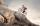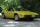Locomotive

Locomotive pulled a train permanent load of 200 kN horizontally along the track of 5km. What work it done?

Result

W =  1000 MJ

Solution:Leave us a comment of example and its solution (i.e. if it is still somewhat unclear...):Be the first to comment!To solve this example are needed these knowledge from mathematics:

Do you want to convert length units?

Next similar examples:

1. Volleyball8 girls wants to play volleyball against boys. On the field at one time can be six players per team. How many initial teams of this girls may trainer to choose?
2. Theorem proveWe want to prove the sentence: If the natural number n is divisible by six, then n is divisible by three. From what assumption we started?
3. Average speed 4Starting at home Tony traveled uphill to the store for 45 minutes at 8 miles per hour. he then traveled back home on the same path at a speed of 24 miles per hour. what is his average speed for the entire trip?
4. First manWhat is the likelihood of a random event where are five men and seven women first will leave the man?
5. Against each otherFrom two points A, B distant 23 km at the same time started two cars against each other at speeds 41 km/h and 65 km/h. How long does cars meet and what distance passes each of them?
6. One halfOne half of ? is: ?
7. PowersExpress the expression ? as the n-th power of the base 10.
8. Two equationsSolve equations (use adding and subtracting of linear equations): -4x+11y=5 6x-11y=-5
9. Functions f,gFind g(1) if g(x) = 3x - x2 Find f(5) if f(x) = x + 1/2
10. 6 termsFind the first six terms of the sequence. a1 = 7, an = an-1 + 6
11. Factory and divisionsThe factory consists of three auxiliary divisions total 2,406 employees. The second division has 76 employees less than 1st division and 3rd division has 212 employees more than the 2nd. How many employees has each division?
12. Nineteenth memberFind the nineteenth member of the arithmetic sequence: a1=33 d=5 find a19
13. Linear systemSolve a set of two equations of two unknowns: 1.5x+1.2y=0.6 0.8x-0.2y=2
14. TreesA certain species of tree grows an average of 0.5 cm per week. Write an equation for the sequence that represents the weekly height of this tree in centimeters if the measurements begin when the tree is 200 centimeters tall.
15. TrigonometryIs true equality? ?
16. ExaminationThe class is 21 students. How many ways can choose two to examination?
17. ConfectioneryThe village markets have 5 kinds of sweets, one weighs 31 grams. How many different ways a customer can buy 1.519 kg sweets.